Thermodynamics and Propulsion
Subsections

16.5 Steady Quasi-One-Dimensional Heat Flow in Non-Planar Geometry

The quasi one-dimensional equation that has been developed can also be applied to non-planar geometries, such as cylindrical and spherical shells.

16.5.1 Cylindrical Shell

An important case is a cylindrical shell, a geometry often encountered in situations where fluids are pumped and heat is transferred. The configuration is shown in Figure 16.10.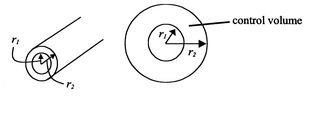For a steady axisymmetric configuration, the temperature depends only on a single coordinate () and Equation (16.13) can be written as(16..25)

or, since,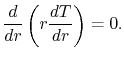(16..26)

The steady-flow energy equation (no fluid flow, no work) tells us that, or(16..27)

The heat transfer rate per unit length is given by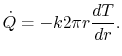(16..28)

Equation (16.26) is a second order differential equation for. Integrating this equation once gives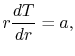(16..29)

whereis a constant of integration. Equation (16.29) can be written as(16..30)

where both sides of Equation (16.30) are exact differentials. It is useful to cast this equation in terms of a dimensionless normalized spatial variable so we can deal with quantities of order unity. To do this, divide through by the inner radius,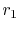,(16..31)

Integrating (16.31) yields(16..32)

To find the constants of integrationand, boundary conditions are needed. These will be taken to be known temperatures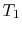andatandrespectively. Applying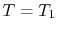at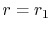gives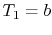. Applying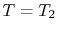atyieldsorThe temperature distribution is thus(16..33)

As said, it is generally useful to put expressions such as (16.33) into non-dimensional and normalized form so that we can deal with numbers of order unity (this also helps in checking whether results are consistent). If convenient, having an answer that goes to zero at one limit is also useful from the perspective of ensuring the answer makes sense. Equation (16.33) can be put in nondimensional form as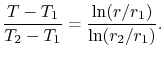(16..34)

The heat transfer rate,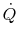, is given by(16..35)

per unit length. When the heat flow rate is written so as to incorporate our definition of thermal resistance,comparison with (16.35) reveals the thermal resistanceto be(16..36)

The cylindrical geometry can be viewed as a limiting case of the planar slab problem. To make the connection, consider the case when. From the series expansion for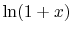we recall that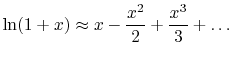(16..37)

(Look it up, try it numerically, or use the binomial theorem on the series (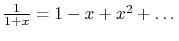) and integrate term by term.)

The logarithms in Equation (16.34) can thus be written as(16..38)

and(16..39)

in the limit of. Using these expressions in Equation (16.33) gives(16..40)

With the substitution of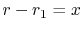, andwe obtain(16..41)

which is the same as Equation (16.19). The plane slab is thus the limiting case of the cylinder if, where the heat transfer can be regarded as taking place in (approximately) a planar slab.

To see when this is appropriate, consider the expansion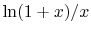, which is the ratio of heat flux for a cylinder and a plane slab.

For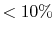error, the ratio of thickness to inner radius should be less than 0.2, and for 20% error, the thickness to inner radius should be less than 0.5 (Table 16.2).

Table 16.2: Utility of plane slab approximation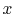0.1 0.2 0.3 0.4 0.5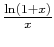0.95 0.91 0.87 0.84 0.81

16.5.2 Spherical Shell

A second example is the spherical shell with specified temperatures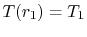and, as sketched in Figure 16.11.The area is now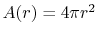, so the equation for the temperature field is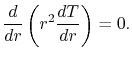(16..42)

Integrating Equation (16.42) once yields(16..43)

Integrating again gives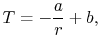(16..44)

or, normalizing the spatial variable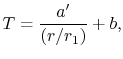(16..45)

where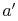andare constants of integration. As before, we specify the temperatures atand. Use of the first boundary condition gives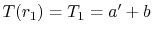. Applying the second boundary condition gives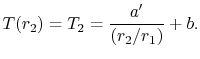Solving forand,(16..46)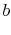(16..47)

In non-dimensional form the temperature distribution is thus(16..48)

UnifiedTP